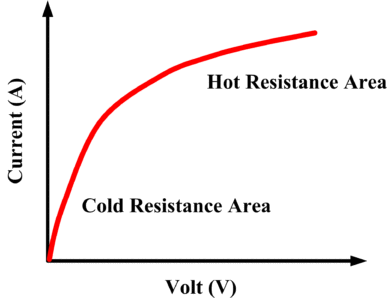Home / Basic Electrical / Nonlinear Resistors | Characteristics Curves of Nonlinear Devices

# Nonlinear Resistors | Characteristics Curves of Nonlinear Devices

Want create site? Find Free WordPress Themes and plugins.

In most circuits, we can assume that resistance is constant in relation to current and voltage. This linear relation can be graphically shown in figure 1.Fig.1: Plot of Linear Relation between Current and Voltage

For example, if 3V is applied to a certain resistor and 1A flows, then 6V applied to the same resistor will cause double the current, or 2A, to flow.

## Nonlinear Resistance

The property exhibited by devices whose resistance does not change uniformly with changes in voltage or current.

Many different devices have characteristic curves (I-V plots) that are not straight lines, and they are, therefore, not linear devices. Such devices are called nonlinear devices since their resistances are nonlinear resistances.

Typical nonlinear devices are the filament of an incandescent lamp, diode, thermistor, and varistor (a special resistor made of Carborundum crystals held together by a binder). The curve in figure 2 shows how the current through a varistor increases very rapidly when the voltage across the device increases beyond a certain amount (about 100 V in this case). A corresponding rapid decrease in resistance also occurs. Varistors are used to provide overvoltage protection in certain circuits.Fig.2: Varistor I-V Curve

A thermistor is another special resistor. It is made of metallic oxides in a suitable binder and has a large negative coefficient of resistance (that is, resistance goes down with an increase in temperature). Figure 3 illustrates this resistance-temperature relationship.Fig.3: Thermistor resistance-temperature relationship

The curve in figure 4 shows how the resistance changes with voltage for an incandescent lamp.Fig.4:  Incandescent Lamp I-V Curve

Figure 5 shows the I-V characteristic of a typical silicon diode.Fig.5:  Silicon Diode I-V Curve

The important thing to remember about any nonlinear resistance is that the resistance calculated for one value of V and I cannot be used when V and I change. But by making a number of changes in voltage and current, we can develop a resistance curve like the curves in above figures.

Did you find apk for android? You can find new Free Android Games and apps.